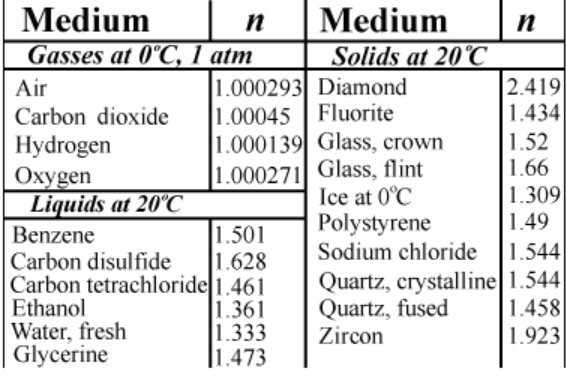# Problem: Suppose the speed of light in a particular medium is 2.012 times 108 m/s. Part (a) Calculate the index of refraction for the medium. Part (b) Based on the table, identify the most likely substance.

###### FREE Expert Solution

Part (a)

Refractive index, η:

$\overline{){\mathbf{\eta }}{\mathbf{=}}\frac{\mathbf{c}}{\mathbf{v}}}$

92% (137 ratings)###### Problem Details

Suppose the speed of light in a particular medium is 2.012 times 108 m/s.Part (a) Calculate the index of refraction for the medium.

Part (b) Based on the table, identify the most likely substance.